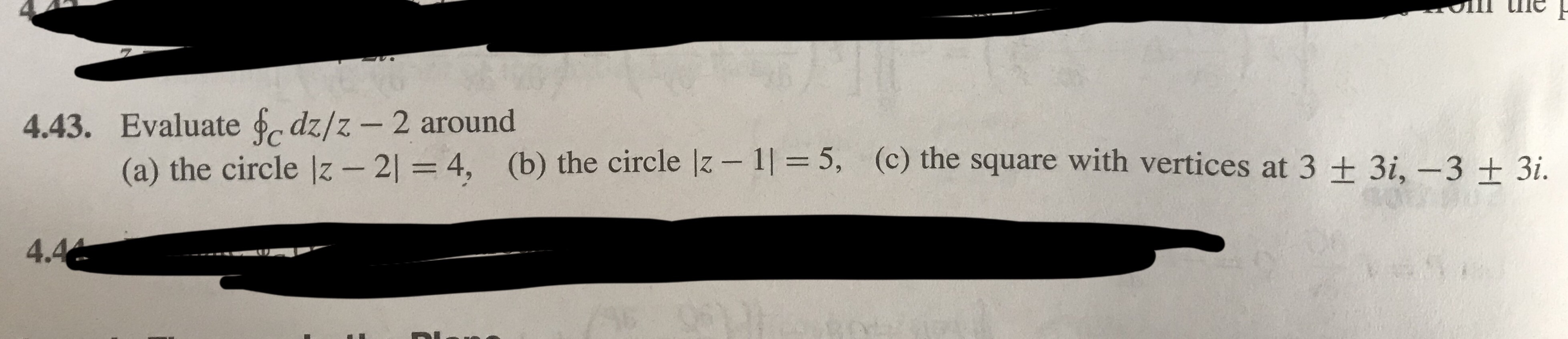# In tie4.43. Evaluate ⓑndz/2-2 around(a) the circle z2 = 4,(b) the circleII = 5,(c) the square with vertices at 3 + 3,3 ± 3il.4.4

Question
3 views

Z is a complex variable, z=x+iy

Question attached in photohelp_outlineImage TranscriptioncloseIn tie 4.43. Evaluate ⓑndz/2-2 around (a) the circle z 2 = 4, (b) the circle II = 5, (c) the square with vertices at 3 + 3, 3 ± 3i l. 4.4 fullscreen
check_circle

Step 1

To evaluate the given integral over three different given contours

Step 2

Points to note: (1) All the integrals have the same value ; (2) they can be evaluated simply by applying residue formula (no need to calculate in a tedious manner)

Step 3

All the three contours are sketched in the diagram. Note all of contain the point z=2 , which is the only pole for the integrand. As per Cauchy's residue formula , ...

### Want to see the full answer?

See Solution

#### Want to see this answer and more?

Solutions are written by subject experts who are available 24/7. Questions are typically answered within 1 hour.*

See Solution
*Response times may vary by subject and question.
Tagged in

### Math